## Friday, May 30, 2014

### Understanding Linear Regression and Code in R

What is actually Regression?

Regression is the attempt to establish a mathematical relationship between variables.
It can be used to extrapolate or to predict the value of 1 variable from other given variables.

Like - By collection Flood data , we can calculate the prediction of flood each year .

so for the basic formula of Linear Relationship-

y = b + mx

where x is an independent variable
y is a dependent variable
m is the slope

And we are trying to predict the value of Y from given x

"Correlation" measures the dependability of the relationship (the goodness of fit of the data to the mathematical relationship). It is a measure of how well one variable can predict the other (given the context of the data), and determines the precision you can assign to a relationship

NOTE : Alternative names for independent variables (especially in data mining and predictive modeling) are input variables, predictors or features. Dependent variables are also called response variables, outcome variables, target variables or output variables. The terms "dependent" and "independent" here have no direct relation to the concept of statistical dependence or independence of events.

THE COFFICIENT

==============

The easiest thing to understand here is if X value is .65 standard deviation(a quantity expressing by how much the members of a group differ from the mean value for the group.) units from the mean, then its y pair should also be .65 standard deviatuon units from the mean if THERE IS A PERFECT FIT.

In a perfect fit a y value's position in its distribution will be in the same position as its corresponding x value' position in its distribution - a one to one correspondence

The correlation coefficient measures only the degree of linear association between two variables

LINEAR MODEL

============

The learning algorithm will learn the set of parameters such that

the sum of square error (yactual - yestimate)2 is minimized.

Here I tried multiple Linear Model (lm) operation on the set of "datasets" , and worked on the example of iris-

 plot(iris$Petal.Length,iris$Petal.Width, col = c("red","green","blue")[unclass(iris$Species)],pch = c(15,24,18)[unclass(iris$Species)] , main = "Petal Length vs Weight")
legend("topleft", pch = c(15,24,18),col = c("red","green","blue"),legend = c("setosa","versicolor","virginica"))
lm_petal <- lm(iris$Petal.Width~iris$Petal.Length);
abline(lm_petal$coefficients,col = "black") summary(lm_petal)  on Running the above , I got my lm output. well now output was a big big mystery for me as I actually needed to understand what all those details data about , and since I am anyway working on data analysis , it became my responsibility to understand the output. So here my output looks like a devil to me .. I mean some nightmare mathematical buzzword ..Plot of LMLinear Model Output So from "Plot of LM" its quite clear that our black straight line passed through almost all the data and linearly we did quite well, How can say that , because straight black line passed through each of the different data types and all the points are closure to those points and that helped a lot. To answer that we need to understand some of extremely basic and super important points in Data Statistics, yeah I used the work Statistics because this is mathematics . STANDARD DEVIATION - In simple straightforward way, Standard Deviation is the measure of how spread the numbers are. Read more about the same Standard Deviation "Lower value of Standard Deviation" means all the data points are close to mean and higher means all data points are scattered everywhere" Now From the output , Residuals are nothing but "A statistical term used to describe the standard deviation of points formed around a linear function, and is an estimate of the accuracy of the dependent variable being measured. " COEFFICIENTS: Each coefficient in the model is a Gaussian (Normal) random variable. The βi^ is the estimate of the mean of the distribution of that random variable, and the standard error is the square root of the variance of that distribution. It is a measure of the uncertainty in the estimate of the βi t-valueThe t statistics are the estimates (βi^) divided by their standard errors (σi^). Simple words t value is the value of the t-statistic for testing whether the corresponding regression coefficient is different from 0. p-valueThe p-value is an estimate of the probability of seeing a t-value as extreme, or more extreme the one you got, if you assume that the null hypothesis is true (the null hypothesis is usually "no effect", unless something else is specified). So if the p-value is very low, then there is a higher probability that you're seeing data that is counter-indicative of zero effect. In other situations, you can get a p-value based on other statistics and variables. More on p-value - p-value Residual Standard Error -The residual standard error is an estimate of the parameter σ. The assumption in ordinary least squares is that the residuals are individually described by a Gaussian (normal) distribution with mean 0 and standard deviation One more point the stars in output summary shows the significance of those features to calculate the result and if there is no star (*) , you can ignore. That's enough, we got lot more details now lets move on to the actual picture, so from our output it can derive that our lm is close to datasets and it will give us proper result. Now in the same set of data , I thought of running classification algorithm using lm , I mean I am trying to derive the result as classification of "isSetosa" . For doing this 1st I divide my data into training set and test set, then I added a "setosa" check column in my traindata set and ran lm-  testData <- which(1:length(iris[,1])%%5 == 0) traindata <- iris[-testData,] testdata <- iris[testData,] lm_petal <- lm(Petal.Width ~ Petal.Length,data = traindata); prediction <- predict(lm_petal,testdata,type='response') round(prediction,3) cor(prediction,testdata$Petal.Width);
#Trying to run classification algorithm in the iris example
#Add a New column to find Binary Classification
newCol <- data.frame(isSetosa = (traindata$Species == "setosa")) #Appending the Column to the existing iris iris_new <- cbind(traindata,newCol) head(iris_new) formula <- isSetosa ~ (Sepal.Length + Sepal.Width +Petal.Length+Petal.Width); lm_new_out <- lm(formula,data = iris_new) summary(lm_new_out) lm_new_out <- predict(lm_new_out,testdata) cor(prediction,testdata$Petal.Length);
cor(prediction,testdata$Petal.Width); cor(prediction,testdata$Sepal.Length);
cor(prediction,testdata$Sepal.Width); round(prediction_new,1)  So on running the same , I got this output - So from the above output , we can see 3 start(*) and ie 1 * for Sepal.Length , 4 * for other2 and no star for Sepal.width. To generalize the same I ran cor on the same features and Corelation as well gave me the same result. and To find the rightness of the model I ran the prediction on the model and found Trained Output as a superb output. σ ## Wednesday, May 28, 2014 ### Exploratory Data Analysis Basics ## Principles of Data Analysis Principle 1 - - Always try to find a hypothesis which can be compared with other hypothesis. So point comes here is "compared to what ? ". Principle 2 - - Needs to justify the cause of framework we derive Principle 3 - -Show Multivariate data or more that one dimension of data , to precisely justify your point . As data always speak and more and more data with proper representation will prove that point.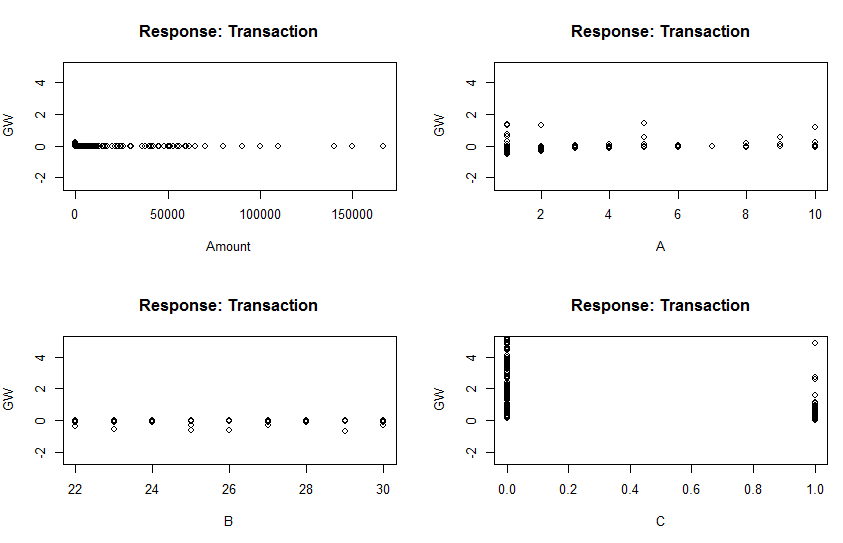Multivariate Data Representation So this kind of graphical representation speak about generalized weight of each of the feature parameter in neural network, so multivariate data representation does precisely show the representation. Principle 4 - - Integration of Evidence, It means always try to show almost all dimension of data not just limited. Add words , numbers , images or almost everything you have to show the data . So main idea is its you who drive the data to represent, not the tool which just takes the data and plot something random. Principle 5 - -Sources where the data came from , so kind of evidence of your plot.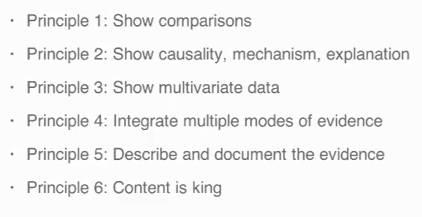Main Principles of Exploratory Data Analysis ### Why Exploratory Data Analysis • Because they can be made easily and faster • Can help for personal understanding • Explain how data look like which is the main important for any Data • Look and Feel is NOT the primary concern about exploratory data analysis ### Example of Exploratory Data Analysis I have my Bank account details and I needed to find out is there any time when I crossed more than 80000 from my Bank account transaction in terms of Debit . First I'll be working on 1-Dimensional Plots like Box , Hist & Bar So now I am assuming or trying to show my data as Categorical Variable. DataSet Download So my data looks like above. First I wanted to plot a Boxplot on my Amount data to check the density of data with median and mean  data <- read.csv("D:/tmp/mlclass-ex1-005/mlclass-ex3-005/R-Studio/account.csv") head(data) #Replace the comma from Amount data$Amount <- as.numeric(gsub(",", "", gsub("", "", data$Amount)))   #Plotting the Box Plot boxplot(data$Amount , col = "yellow")
#This corresponds that almost majority of my data is below 8000 , very less population around 80000
abline( h = 80000) #h = horizental


Well I realized that its useless for me as I am not even able to derive anything from this plot.

So now I decided to go ahead to some better way like to draw a histogram with rug and found out -

 #Plotting Histogram of the data to get more details about the data
hist(data$Amount , col = "light green" ) #rug shows exactly where the points are rug(data$Amount)
#rug shows that bulk of the data is in between 25000 itself


Yeah this seems to better but I can make it more better by finding Median and Mean over that same graph and bring some more break points-

 #Adding breaks add more number of plots or break the graphs in smaller parts
hist(data$Amount , col = "light blue" ,breaks = 20) abline( v = 85000, lwd = 4) #v = vertical #Since hist doesn;t show median so that as well can be added here abline( v = median(data$Amount) , col = "red" , lwd = 4)


Great , now I have something to derive on my Data.

But how about trying the same with other feature like Transaction as I have binary value of it ie 1 for Debit or 0 for Credit.

Box Plot - a horrible mistake

Histogram , well it gives something but its not meant for this-

And finally here we find barplot is the best suit which actually can gives us the frequency of 0 or 1  , we can derive the occurrences on my credit account which is extremely poor . :(

 #Trying to Plot histogram of Transaction by changing to numeric
data$Transaction <- as.numeric(data$Transaction=="Dr.")
#You won't get any details from Box Plot of Transaction because you have just 2 entries
boxplot(data$Transaction , col = "yellow") #Here we can derive the frequency of 0 and 1 hist(data$Transaction , col = "light green")
#Bar plot will be best suit for finding the majority of Debit and Credit from your account
barplot(table(data$Transaction) , col = "light blue" , main = "Ration of Credit vs Debit ")  Now to work mainly on 2-Dimensional plots like Scatter-plot Well Here I just wanted to find what is the density of Debit and Credit for my account Transaction and I was surprised to see its "Credit" For the same purpose , Histogram Representation where its more cleared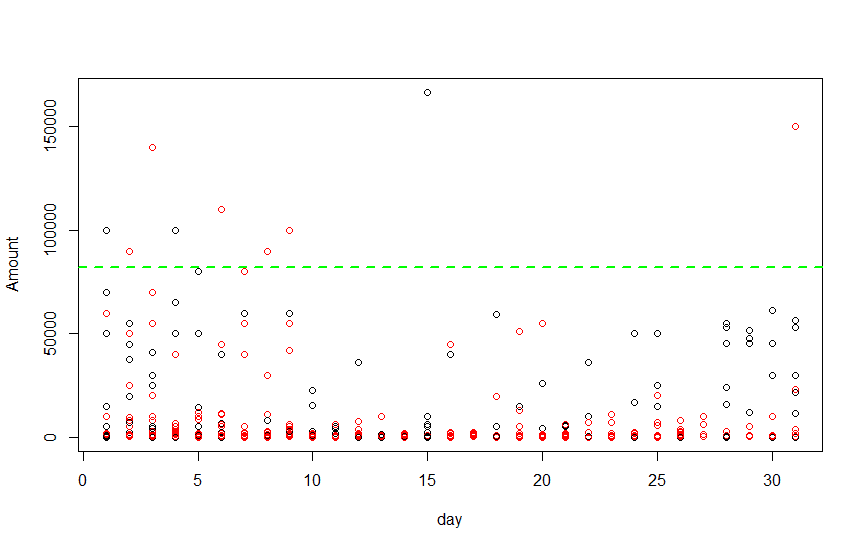Amount vs Days spending and Trying to find Debit or Credit on top of it  data <- read.csv("D:/tmp/mlclass-ex1-005/mlclass-ex3-005/R-Studio/account.csv") head(data) #Replace the comma from Amount data$Amount <- as.numeric(gsub(",", "", gsub("", "", data$Amount))) #Plotting 2-Dimensional Using Box Plot #Trying plot Amounts based on Credit or Debit boxplot(Amount ~ Transaction , data = data , col = "red") #Credit is More than Debit , hows that possible , that is because I am comparaing # based on the amount not with frequency , so amount speaks a total different chapter #Histogram representation for the same purpose hist(subset(data,Transaction == "Dr.")$Amount, col = "green" , breaks = 20)
hist(subset(data,Transaction == "Cr.")$Amount, col = "green" , breaks = 20) #data$Transaction <- as.numeric(data$Transaction=="Dr.") #ScatterPlot #Split Date in Day Month & year data$Transaction.Date <- as.Date(data$Transaction.Date, format="%d/%m/%Y") month = as.numeric(format(data$Transaction.Date, format = "%m"))
day = as.numeric(format(data$Transaction.Date, format = "%d")) year = as.numeric(format(data$Transaction.Date, format = "%Y"))
#Shows the Amount transaction on Each day , and col sepeartes the value based on Dr. or Cr. type
with(data,plot(day,Amount, col = Transaction)) #col will take Dr. as color red
abline( h = 82000, lwd = 2, lty = 2, col = "green")
#points(data$Amount,data$Transaction == "Dr.",col = "blue")
#points(data$Amount,data$Transaction == "Cr.",col = "red")
#Seperate the data based on year
#with(data,plot(day,Amount, col = Year))
#MULTIPLE SCATTERPLOT like I Did in Histogram
s1 <- subset(data,Transaction == "Dr.")
s2 <- subset(data,Transaction == "Cr.")
with(s1,plot(c(1:nrow(s1)),Amount,main = "Debit"))
#day is not equal to size of x , so we need to find
with(s2,plot(c(1:nrow(s2)),Amount,main = "Credit"))


So whenever the data comes, proper basic visualization is going to solve most of the trouble and that is what we did and that is called Exploratory Data Analysis.
We got the preview of data , and almost tried to follow the principles... is it .. have I actually followed all the principles....... :)

So its a kind of quick and dirty approach to summarize the data and it just ease our job to decide the model and strategy for the next step.

## Tuesday, May 27, 2014

### The Problem posted previously neural not respond I tried to find the problem based on actual data-

Now First I tried to run the logic on existing set of data -

 library("neuralnet")
setClass("myDate")
#Replace the comma from Amount
data$Amount <- as.numeric(gsub(",", "", gsub("", "", data$Amount)))
#Change Dr.(1) and Cr.(0)
data$Transaction <- as.numeric(data$Transaction=="Dr.")
#Split Date in Day Month & year
data$Transaction.Date <- as.Date(data$Transaction.Date, format="%d/%m/%Y")
month = as.numeric(format(data$Transaction.Date, format = "%m")) year = as.numeric(format(data$Transaction.Date, format = "%Y"))


And the data looks like-

For this data I already provided different plots based on different perm comp here Previous

And as we know we get a huge Error rate after running the neural network algorithm -

 output <- neuralnet(Transaction ~ Amount+month,data,hidden = 4,threshold = 0.01,linear.output=FALSE, likelihood=TRUE)
print(output$result) plot(output,rep = "best")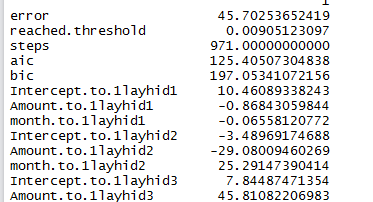I am going to present an another way of visualizing the result. Visualize the result from using generalized Weights gwplot uses the calculated generalized weight provided by nn$generalized.weights

 out <- cbind(output$covariate,output$net.result[])
dimnames(out) <- list(NULL, c("Amount","Month","nn-output"))


 #Plotting Generalized weight
#The distribution of the generalized weights suggests that the covariate Amount
#has no effect on the case-control status since all generalized weights are nearly zero
par(mfrow=c(2,2))
gwplot(output,selected.covariate="Amount", min=-2.5, max=5)
gwplot(output,selected.covariate="month", min=-2.5, max=5)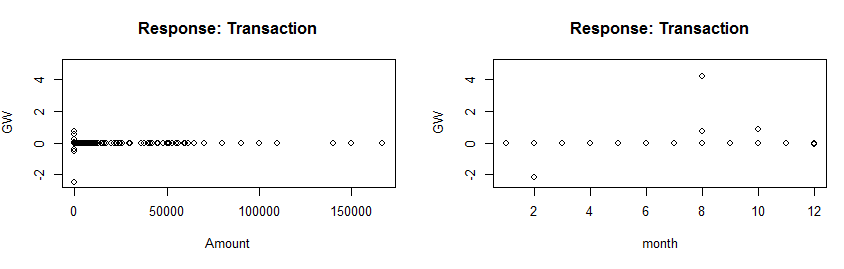The distribution of the generalized weights suggests that the covariate Amount has no effect on the case-control status since all generalized weights are nearly zero

### I Added few features in my Data Set with some sense and again did all these steps

 library("neuralnet")
data_new <- data;
data_new[c("A","B","C")] <- NA
data_new$A <- sample(1:10,nrow(data_new),replace = TRUE) data_new$B <- sample(22:30,nrow(data_new),replace = TRUE)
data_new$C <- as.numeric(data_new$Transaction =="1")


And after running rest of the code,

 plot(data_new$Amount, data_new$A+data_new$B+data_new$C, main="Transaction vs Amount",
xlab="Amount", ylab="A+B+C", pch=1, col="red")
output_new <- neuralnet(Transaction ~ Amount+A+B+C,data_new,hidden = 4,threshold = 0.01,linear.output=FALSE, likelihood=TRUE)
print(output_new$result) plot(output_new,rep = "best") #How well my Data Fits Here out_new <- cbind(output_new$covariate,output_new$net.result[]) dimnames(out_new) <- list(NULL, c("Amount","A","B","C","neural-output")) head(out_new) par(mfrow=c(2,2)) #two covariates A and C have a nonlinear effect since #the variance of their generalized weights is overall greater than one gwplot(output_new,selected.covariate="Amount", min=-2.5, max=5) gwplot(output_new,selected.covariate="A", min=-2.5, max=5) gwplot(output_new,selected.covariate="B", min=-2.5, max=5) gwplot(output_new,selected.covariate="C", min=-2.5, max=5)  I got an excellent Error rate as well as distribution of the generalized weights were good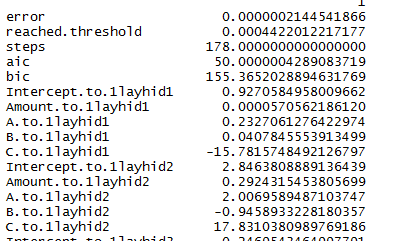two covariates A and C have a nonlinear effect since the variance of their generalized weights is overall greater than one ### Neural Network – Where it can’t give any proper Output ## Neural Network – Where it didn’t produce any output I wanted to analyze my expenses so I found statistical analysis on my expense report will definitely going to help there. So I buckled up and downloaded the previous 4 years statement of my bank, and the following kind of data I found – Great so now I am ready and thought lets first draw some graphs from the above dataset. First thing I found is month wise expenses and I got – s Great, I just found that I am doing transaction almost every month and as the amount increases, density of the data is getting decreases, naturally as I don’t have that much money to do the transaction. Now you might find some lower Green colored data, well that is a trouble that shows my debit, so almost whatever the amount credits , I just debit , so you can assume my great bank balance. So now I thought of shuffling through the same data in yearly pattern- Almost similar result. Now the point comes , on which data and what kind of classification output I am expecting to run Neural Network. First I thought based on my amount input , lets classify it will be Debit(1) or Credit(0). Uhhh ,.. some illogical point but I just wanted to see what happens- I ran the neural network and this is the result I got- output <- neuralnet(Transaction ~ Amount,data,hidden = 10,threshold = 0.01,linear.output=FALSE, likelihood=TRUE) Yeah Yeah .. Error is too high , well but the formula is pretty straightforward. Then I realize, my formula doesn’t make any sense at all. As my Debit and Credit both are in sync and even manually I can’t justify the logic that a certain amount is Debit or Credit. And the solution I found by adding new features Add new features in Neural and Test SOURCE CODE  library("neuralnet") # create a class setClass("myDate") setAs("character", "CrDr", function(from) c(Cr.=1,Dr.=0)[from]) setAs("character","myDate", function(from) as.Date(from, format="%d/%m/%Y") ) data <- read.csv("D:/tmp/mlclass-ex1-005/mlclass-ex3-005/R-Studio/account.csv") head(data) #Replace the comma from Amount data$Amount <- as.numeric(gsub(",", "", gsub("", "", data$Amount))) #Change Dr.(1) and Cr.(0) data$Transaction <- as.numeric(data$Transaction=="Dr.") data$Transaction.Date <- as.Date(data$Transaction.Date, format="%d/%m/%Y") month = as.numeric(format(data$Transaction.Date, format = "%m"))
year = as.numeric(format(data$Transaction.Date, format = "%Y")) head(data) plot(data$Amount, year, main="Amount vs Year",
xlab="Amount", ylab="Year", pch=10, col="blue")
axis(2, at=x,labels=x, col.axis="red", las=2)
plot(data$Amount, month, main="Amount vs Month & Dr.", xlab="Amount", ylab="Month", pch=1, col="red") points(data$Amount, data$Transaction==1, pch=5,col = "green", cex = 1.0) plot(data$Transaction, data$Amount, main="Transaction vs Amount", xlab="Transaction", ylab="Amount", pch=1, col="red") #Month wise Credit & Debit #To convert Dr. Cr. of Transaction Type to 1 for Dr. and 0 for Cr. output <- neuralnet(Transaction ~ Amount,data,hidden = 4,threshold = 0.01,linear.output=FALSE, likelihood=TRUE) print(output$result)
plot(output,rep = "best")
testdata <- c(1000,2000,12000,45001,19123,36000)
#test_sample <- subset(testdata,select = c("day","month","year"))
result <- compute(output,testdata);
print(round(result\$net.result))